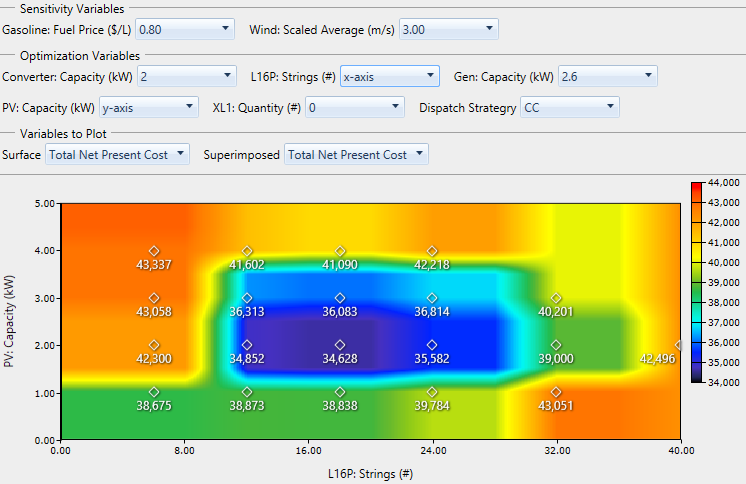# HOMER Pro 3.9

 Navigation: Results > Optimization Results Graphical ModeThe Optimization Results page shows calculation results in tables, or Tabular mode, by default. To change this presentation to show the data in graphs, check the Graphical radio button.

The Graphical Results page contains several plot types that allow you to visualize the results in various ways. You can select the plot type you want to see from the OptimalSystemTpyePlot drop-down menu beneath the Tabular and Graphical radio buttons. The first four options, the Optimal System Type Plot, Surface Plot, Line Plot, and Spider Plot provide a graphical representation across the sensitivity results. See 3.3 Sensitivity Results for more information on the first four options.

The last two plots, the Optimization Plot and the Optimization Surface Plot allow you to visualize the optimization for the sensitivity case you selected.

## Optimization Plot

The Optimization Plot shows each simulation as a single point on a set of axes you can choose. First, choose which sensitivity case to view from the Sensitivity Variables drop-down menus. Then choose which Optimization Variables you want to see. By default, All is selected for all of the optimization variables, so you see a dot for every simulation that was feasible for this sensitivity case.

The image below is a sample from the Sample-OffGridHouseInMontana.homer file, which is available from the Samples section of HOMER's file menu. The x-axis represents Total Capital Cost, and the y-axis represents the Total Net Present cost. The winning system for this sensitivity case is the lowest dot on the plot, corresponding to the lowest net present cost. This system has a capital cost of \$5,040 and a net present cost of \$24,963. This system is a good balance of capital cost and operating cost, which results in the lowest net present cost in this scenario.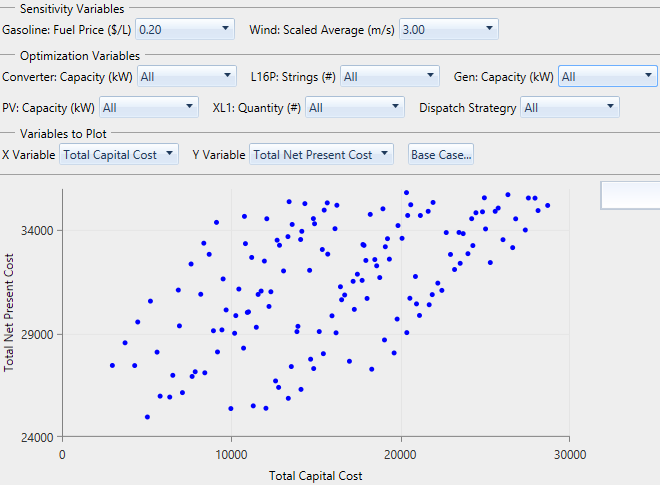You can filter and group the points in this plot by changing the settings in the drop-down menus for each variable under the Optimization Variables section. In the plot below, the generator size (Gen: Capacity) is 2.6 and the Converter: Capacity is Optimal. The graph shows a point for every combination of batteries (L16P: Strings), wind turbines (XL1: Quantity), and PV: Capacity. For each of these combinations (i.e., 6 batteries, 1 kW of PV, and 2 wind turbines), HOMER only uses systems with the 2.6 kW generator (all the zero-size generator systems are infeasible anyway) and the optimal converter size for that case.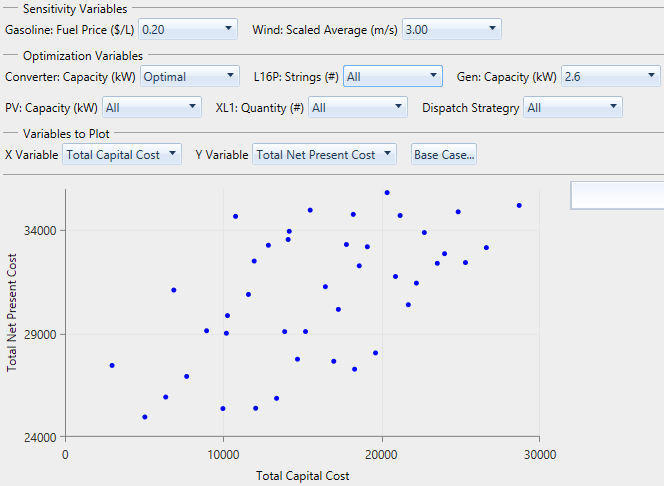In the next image, the storage (L16P: Strings) is Line Series. You can only select Line Series for one of the variables at a time. With this configuration, there is a line for each combination of PV: Capacity and wind turbine (XL1: Quantity) quantity. At one end of each line is the minimum quantity of batteries (zero). At the other end is the maximum number of batteries. Each line is a different color, corresponding to the legend entry which lists the PV: Capacity and XL1: Quantity for that line. You can hover your mouse over any point to see the system architecture associated with it.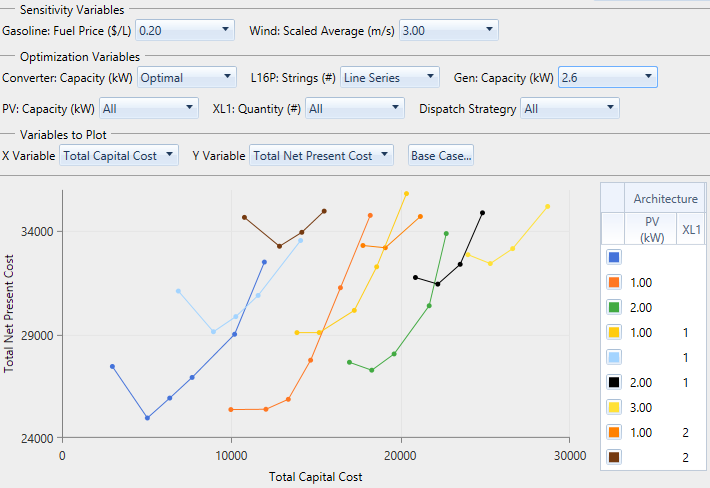Instead of the total 18 lines (representing six sizes of PV and three quantities of wind turbine), there are only ten because when HOMER runs any optimization, it only saves the 2,000 best (lowest NPC) simulation results by default. You can change this default using the Settings option in the File menu. For a large Search Space, as shown in the "Off-grid house in Montana" example, some simulations are left out from the plot, unless you increase the number of simulations to save. HOMER always chooses which simulations to save by NPC, so only poor systems (according to NPC) are discarded. You can be confident that the optimization plot includes the most important simulations, but some trivial systems might be left off.

The Base Case... button, to the right of the X Variable and Y Variable drop-down menus, allows you to choose a single simulation for comparison. This is required to compute some economic quantities. Outputs marked with (*) in the X Variable and Y Variable drop-down menus require you to select a Base Case. After you choose a Base Case, a text summary of the selection appears to the right of the Base Case... button.

## Optimization Surface Plot

The Optimization Surface Plot allows you to view the way the value of one result variable changes over the range of two Optimization Variables. Typical inputs for this plot are: PV capacity and storage quantity on the x- and y-axes, and Total Net Present Cost as the result variable to plot. Like the Optimization Plot (above), first select the sensitivity case from the Sensitivity Variables drop-down menus. Then choose an optimization variable to plot on the x-axis and one for the y-axis. Choose fixed values for the remaining Optimization Variables. You can only set "x-axis" for one variable, and "y-axis" for another variable. If you make an invalid selection (i.e., selecting "x-axis" for more than one variable), the plot does not refresh until you fix the selection.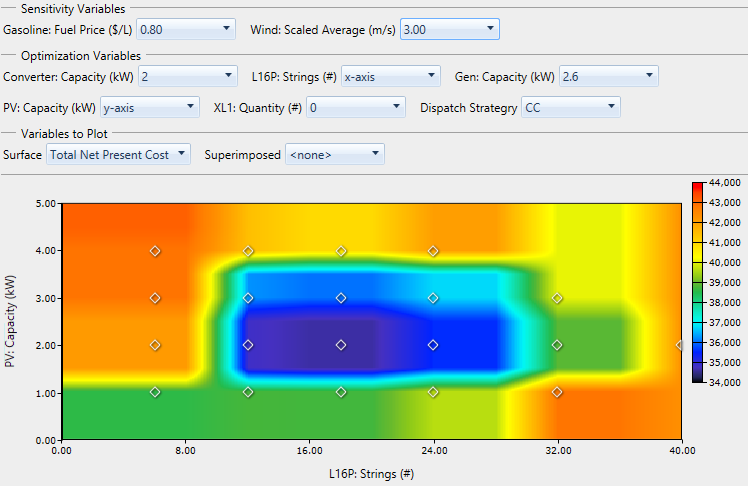The Superimposed drop-down menu under Variables to Plot allows you to choose a second results variable to show on the plot at each point of simulation on the plot. In the image below, Total Net Present Cost is superimposed. This is the same quantity that is drawn as the surface. It lets us see which points were actually simulated, and how the plot interpolates and extrapolates to draw the surface. You can also superimpose a different variable than the one plotted on the surface.